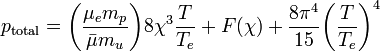User:Tohline/Math/EQ PressureTotal01$~p_\mathrm{total} = \biggl(\frac{\mu_e m_p}{\bar{\mu} m_u} \biggr) 8 \chi^3 \frac{T}{T_e} + F(\chi) + \frac{8\pi^4}{15} \biggl( \frac{T}{T_e} \biggr)^4$